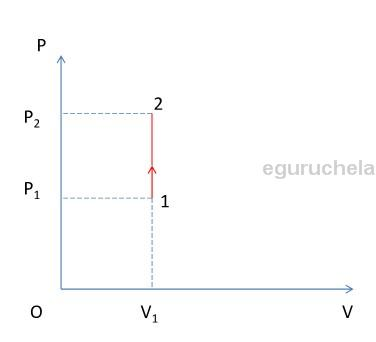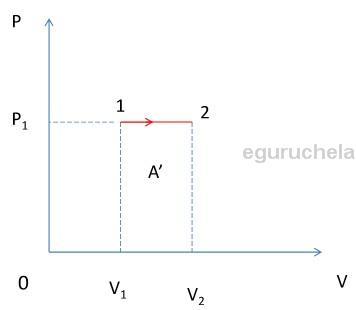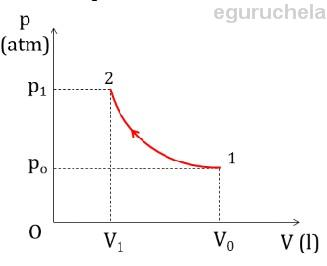# List of formulas related to Thermodynamics

## The First Law Of Thermodynamics

ΔU: change in internal energy, W: work done by the system, Q: heat added into or out of the system

$$\Delta U = W + Q$$

### Application Of First Law To Ideal Gas Laws

Volume is constant

$$\Delta V = 0 => W = 0$$ $$=> Q = \Delta U$$Pressure is constant, W’: work created by gas

$$\Delta V \neq 0 => W \neq 0$$ $$=> Q = \Delta U - W$$ $$W = -W' = p_1(V_2 - V_2)$$ $$=> Q = \Delta U + W'$$Temperature is constant

$$t = costant => \Delta U = 0$$ $$=> Q = \Delta U - W = -W(W \neq 0)$$### The Second Law Of Thermodynamics

The efficiency of a cyclic heat engine

W’: work carried out per cycle(J), Q1: heat absorbed per cycle from the higher temperature(J), Q2’: heat rejected per cycle to the lower temperature(J)

$$H = \frac{W'}{Q_1}$$ $$W' = Q_1 - Q_2')$$ $$=> H = \frac{W'}{Q_1} = \frac{Q_1 - Q_2'}{Q_1}$$

Maximum thermal efficiency

T1,T2: higher and lower temperatures

$$H_{max} = \frac{T_1 - T_2}{T_1}$$